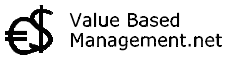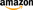## Liquidity RatioThe Cash Ratio (CAR) method is a formula for measuring the liquidity of a company by calculating the ratio between all cash and cash equivalent assets and all current liabilities. It excludes both inventory and accounts receivable in comparison to the Current Ratio. The CAR model measures only the most liquid of all assets against current liabilities, and is therefore seen as the most conservative of the three liquidity ratios. For the Cash Ratio formula, see the picture on the left. This CAR ratio is also known as the Liquidity Ratio and Cash Asset Ratio. The formula is an indicator of the extent to which a company can pay current liabilities without relying on the sale of inventory and without relying on the receipt of accounts receivables. A thing to remember when using the CAR formula is that it ignores timing of both cash received and cash paid out. Book: Steven M. Bragg - Business Ratios and Formulas : A Comprehensive Guide -Book: Ciaran Walsh - Key Management Ratios -Compare also: Current Ratio  |  Z-Score  |  Quick Ratio